## RD Sharma Class 9 Solutions Chapter 1 Number Systems Ex 1.5

These Solutions are part of RD Sharma Class 9 Solutions. Here we have given RD Sharma Class 9 Solutions Chapter 1 Number Systems Ex 1.5

Question 1.
Complete the following sentences:
(i) Every point on the number line corresponds to a … number which many be either … or
(ii) The decimal form of an irrational number is neither … nor …
(iii) The decimal representation of a rational number is either … or …
(iv) Every real number is either … number or … number.
Solution:
(i) Every point on the number line corresponds to a real number which many be either rational or irrational.
(ii) The decimal form of an irrational number is neither terminating nor repeating.
(iii) The decimal representation of a rational number is either terminating or non­terminating, recurring.
(iv) Every real number is either rational number or an irrational number.

Question 2.
Find whether the following statements are true or false:
(i)  Every real number is either rational or irrational.
(ii) π is an irrational number.
(iii) Irrational numbers cannot be represented by points on the number line.
Solution:
(i) True. (Value of π = 3.14)
(ii) False : we can represent irrational number also.

Question 3.
Represent $$\sqrt { 6 }$$, $$\sqrt { 7 }$$, $$\sqrt { 8 }$$ on the number line.
Solution: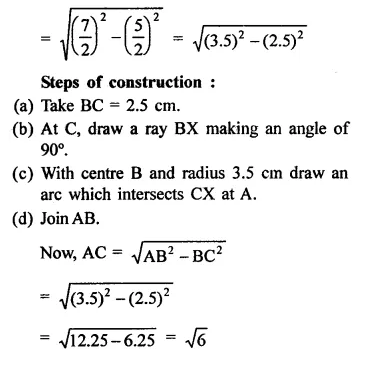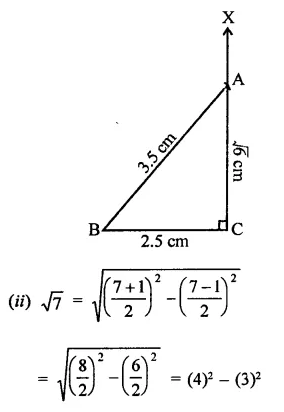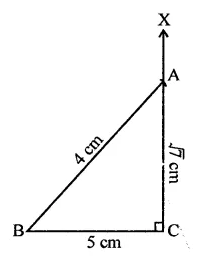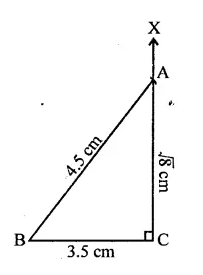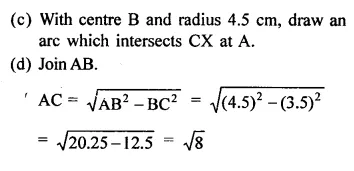Question 4.
Represent $$\sqrt { 3.5 }$$ , $$\sqrt { 9.4 }$$and $$\sqrt { 10.5 }$$ on the real number line.
Solution: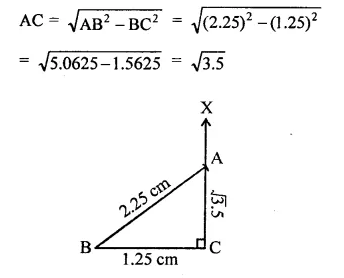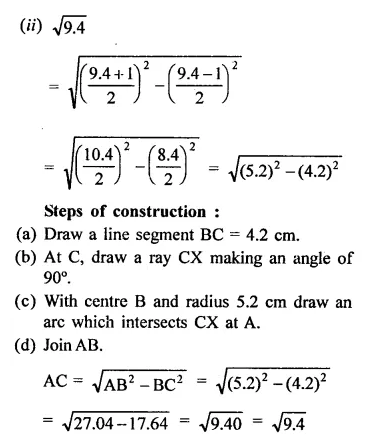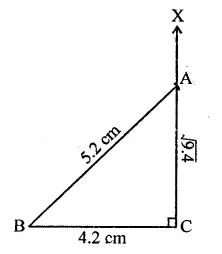Hope given RD Sharma Class 9 Solutions Chapter 1 Number Systems Ex 1.5 are helpful to complete your math homework.

If you have any doubts, please comment below. Learn Insta try to provide online math tutoring for you.